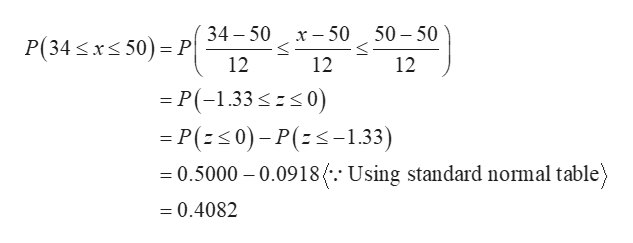# Morgan trucking company determined that on an annual basis the distance traveled per truck is normally distributed with a mean of 50 thousand miles and standard deviation of 12 thousand miles. 1)What the probability that a truck travels less than 34 thousand miles per year?2)What is the probability that a truck travels between 34 and 50 thousand miles per year?3)What is the probability that a truck travel more than 70 thousand miles per yea

Question
49 views

Morgan trucking company determined that on an annual basis the distance traveled per truck is normally distributed with a mean of 50 thousand miles and standard deviation of 12 thousand miles.
1)What the probability that a truck travels less than 34 thousand miles per year?
2)What is the probability that a truck travels between 34 and 50 thousand miles per year?
3)What is the probability that a truck travel more than 70 thousand miles per yea

check_circle

Step 1

It is given that mean and standard deviation are 50 and 12, respectively.

Step 2

Let the variable X denotes the annual basis the distance travelled per truck.

a.

The probability that a truck travels less than 34 thousand miles per year is obtained as follows:

Step 3

b.

The probability that a truck travels between 34 and 50 thousand miles per year is obtained as follows:

...help_outlineImage Transcriptionclose34 50 50 50 х — 50 P(34 xs 50) P 12 12 12 P(-1.33 0) P(s0)-P(-1.33) 0.5000 0.0918 (-.- Using standard normal table = 0.4082 fullscreen

### Want to see the full answer?

See Solution

#### Want to see this answer and more?

Solutions are written by subject experts who are available 24/7. Questions are typically answered within 1 hour.*

See Solution
*Response times may vary by subject and question.
Tagged in

### Data